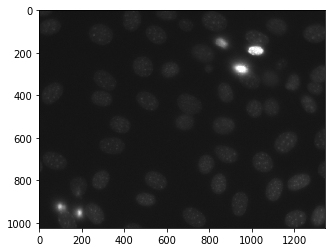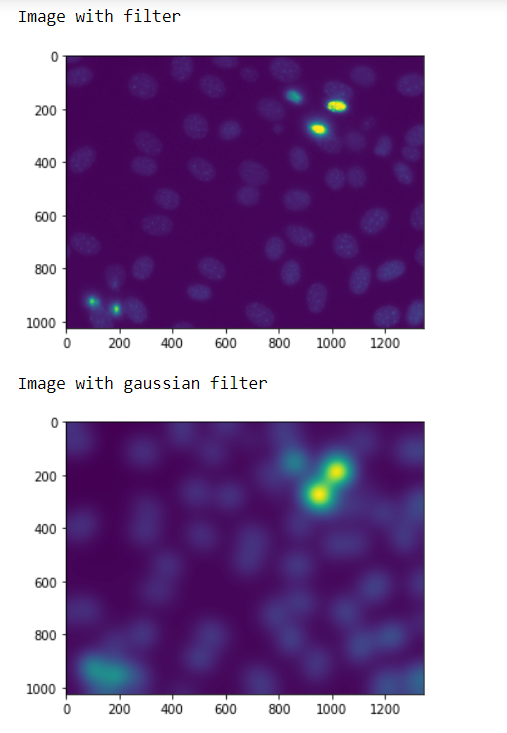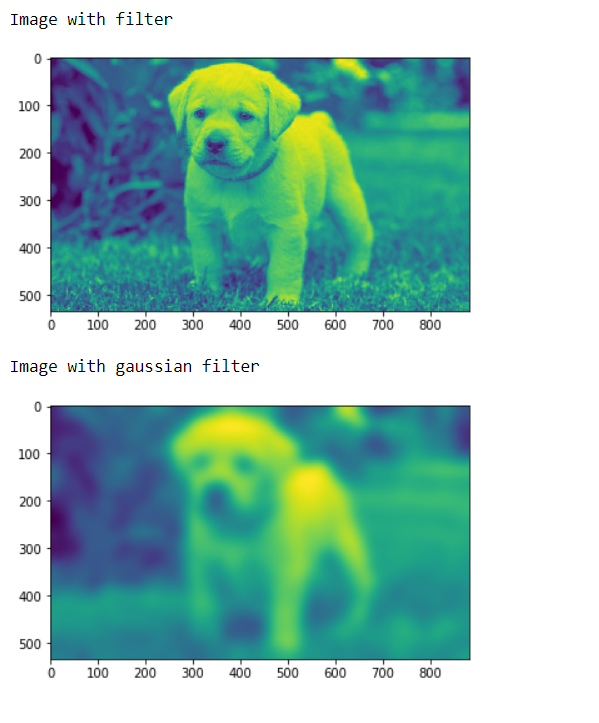# Mahotas – Gaussian filtering

• Last Updated : 06 May, 2021

In this article we will see how we can do Gaussian filtering in mahotas. For this we are going to use the fluorescent microscopy image from a nuclear segmentation benchmark. We can get the image with the help of command given below

`mhotas.demos.nuclear_image()`

A Gaussian filter is a linear filter. It’s usually used to blur the image or to reduce noise. If you use two of them and subtract, you can use them for “unsharp masking” (edge detection). The Gaussian filter alone will blur edges and reduce contrast.
Below is the nuclear_imageIn order to do this we will use mahotas.gaussian_filter method

Syntax : mahotas.gaussian_filter(nuclear, 20)
Argument : It takes numpy.ndarray object as argument and a integer
Return : It returns numpy.ndarray object

Note : The input of the gaussian filter should be the filtered image object
In order to filter the image we will take the image object which is numpy.ndarray and filter it with the help of indexing, below is the command to do this

`image = image[:, :, 0]`

Example 1 :

## Python3

 `# importing required libraries``import` `mahotas``import` `mahotas.demos``import` `numpy as np``from` `pylab ``import` `imshow, show` `# getting nuclear image``nuclear ``=` `mh.demos.nuclear_image()`  `# filtering the image``nuclear ``=` `nuclear[:, :, ``0``]` `print``(``"Image with filter"``)``# showing the image``imshow(nuclear)``show()` `# setting gaussian filter``nuclear ``=` `mahotas.gaussian_filter(nuclear, ``35``)` `print``(``"Image with gaussian filter"``)``# showing the gaussian filter``imshow(nuclear)``show()`

Output :Example 2:

## Python3

 `# importing required libraries``import` `numpy as np``import` `mahotas``from` `pylab ``import` `imshow, show` `# loading image``img ``=` `mahotas.imread(``'dog_image.png'``)` `# filtering the image``img ``=` `img[:, :, ``0``]``  ` `print``(``"Image with filter"``)``# showing the image``imshow(img)``show()`  `# setting gaussian filter``gaussian ``=` `mahotas.gaussian_filter(img, ``15``)` `print``(``"Image with gaussian filter"``)``# showing the gaussian filter``imshow(gaussian)``show()`

Output :My Personal Notes arrow_drop_up